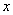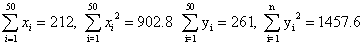Monday 08th August 2022CBSE Guess > Papers > Important Questions > Class XI > 2010 > Maths > Maths By Mr. Anil Kumar Tondak CBSE CLASS XI
Statistics Q.1. Find the mean deviation about the mean for the data: xi : 5 10 15 20 25 f i : 7 4 6 3 5 Q.2. Calculate the mean deviation about median for the following data: Class : 0-10 10-20 20-30 30-40 40-50 50-60 Frequency : 6 7 15 16 4 2 Q.3. Find the mean and variance of first n natural numbers. Q.4. Find the mean and standard deviation using short-cut method: . xi : 60 61 62 63 64 65 66 67 68 f i : 2 1 12 29 25 12 10 4 5 Q.5. The diameters of circles (in mm) drawn in a design are given below: Diameters: 33-36 37-40 41-44 45-48 49-52 No. of circles: 15 17 21 22 25 Calculate the standard deviation and mean diameter of the circles. Q.6. The sum and sum of squares corresponding to length(in cm) and weight y (in gm) of 50 plant products are given below :Which is more varying, the length or weight. Q.7. An analysis of monthly wages paid to workers in two firms A and B, belonging to the same industry, gives the following results: Firm A Firm B No. of wage earners 586 648 Mean of monthly wages Rs 5253 Rs 5253 Variance of the distribution 100 121 of wages (i) Which firm A or B pays larger amount as monthly wages? (ii) Which firm, A or B, shows greater variability in individual wages? Q.8. The variance of 20 observations is 5. If each observation is multiplied by 2, Find the new variance of the resulting observations. Q.9. If each of the observation x1, x2, ...,xn is increased by ‘a’, where a is a negative or positive number, show that the variance remains unchanged. Q.10. The mean and standard deviation of six observations are 8 and 4, respectively. If each observation is multiplied by 3, find the new mean and new standard deviation of the resulting observations. Q.11. The mean and variance of 7 observations are 8 and 16 respectively. If five of the observations are 2, 4, 10, 12, 14. Find the remaining two observations. Q.12. Coefficient of variation of two distributions are 60 and 70 and their standard deviations are 21 and 16 respectively. Find their arithmetic means. Q.13. The mean and standard deviation of 100 observations were calculated as 40 and 5.1 respectively by a student who took by mistake 50 instead of 40 for one observation. What are the correct mean and standard deviation. Q.14. The mean and standard deviation of a group of 100 observations were found to be 20 and 3, respectively. Later on it was found that three observations were incorrect, which were recorded as 21, 21 and 18. Find the mean and standard deviation if the incorrect observations are omitted. Paper By Mr. Anil Kumar Tondak Email Id : [email protected] Ph No.: 9811363962